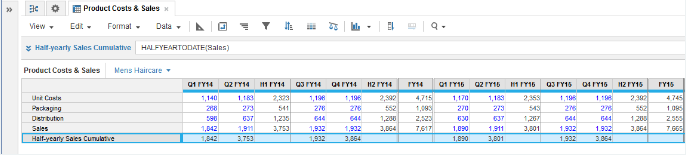1. Calculation functions
2. All Functions
3. Time and Date Functions
4. HALFYEARTODATETakes a single numeric parameter and returns cumulative sums across a half-year time span, and then resets.

## Syntax

`HALFYEARTODATE(x)`

where:

• x: Line item or list property containing the numeric field to summarize

## Format

Input Format Output Format
x: Number Number

## Arguments

The function uses the following arguments:

• x: Number: Numeric line item, property, or expression

## Constraints

The function has the following constraints:

• Time period granularity of source must be Day, Week, Month, or Quarter.
• Cannot be used if model timescale is General Weeks.

## Example

In this example, HALFYEARTODATE is used to show cumulative sales figures for each half-year period for FY14 and FY15:

`HALFYEARTODATE(Sales)`